Courses

# Gaseous State MCQ - 2 (Advanced)

## 15 Questions MCQ Test Chemistry for JEE Advanced | Gaseous State MCQ - 2 (Advanced)

Description
This mock test of Gaseous State MCQ - 2 (Advanced) for JEE helps you for every JEE entrance exam. This contains 15 Multiple Choice Questions for JEE Gaseous State MCQ - 2 (Advanced) (mcq) to study with solutions a complete question bank. The solved questions answers in this Gaseous State MCQ - 2 (Advanced) quiz give you a good mix of easy questions and tough questions. JEE students definitely take this Gaseous State MCQ - 2 (Advanced) exercise for a better result in the exam. You can find other Gaseous State MCQ - 2 (Advanced) extra questions, long questions & short questions for JEE on EduRev as well by searching above.
*Multiple options can be correct
QUESTION: 1

Solution:
QUESTION: 2

Solution:
QUESTION: 3

### In the Figure, isotherms of CO2 at several temperatures near the critical point are shown. At the critical point (critical state), the distinction between the liquid and gaseous states disappear and the density of the gaseous substance is equal to that in the liquid state. For every gas this occurs at specific values of temperature and pressure, called critical temperature and critical pressure respectively. At temperatures and pressures above the critical point value, a gas is said be in a supercritical state. Critical constants are evaluated by solving the Vander W aals equation which is a cubic in volume. The values are Tc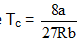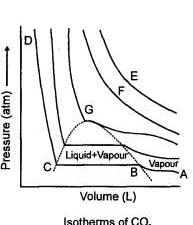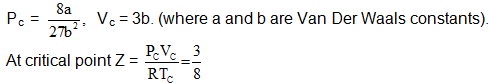In the supercritical region the behaviour is studied by plotting Z vs P plots. The variation in this region is studied with respect to boyles temperature a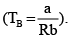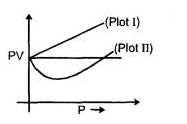At temperature  higher than CB the variation is linear (Plot I) and at temperature lower than CB the variation is non linear (Plot II) With reference to the passage answers the following questions: Q. Two vandaer waals gases have same value of 'a' but different value of b which of the following statement is correct.

Solution:
QUESTION: 4

In the Figure, isotherms of CO2 at several temperatures near the critical point are shown. At the critical point (critical state), the distinction between the liquid and gaseous states disappear and the density of the gaseous substance is equal to that in the liquid state. For every gas this occurs at specific values of temperature and pressure, called critical temperature and critical pressure respectively. At temperatures and pressures above the critical point value, a gas is said be in a supercritical state. Critical constants are evaluated by solving the Vander W aals equation which is a cubic in volume. The values are TcIn the supercritical region the behaviour is studied by plotting Z vs P plots. The variation in this region is studied with respect to boyles temperature aAt temperature
higher than CB the variation is linear (Plot I) and at temperature lower than CB the variation is non linear (Plot II) With reference to the passage answers the following questions:

Q.

Two Vander waals gases have same value 'b' but different 'a' value then which of the following statement is correct under similar condition.

Solution:
QUESTION: 5

In the Figure, isotherms of CO2 at several temperatures near the critical point are shown. At the critical point (critical state), the distinction between the liquid and gaseous states disappear and the density of the gaseous substance is equal to that in the liquid state. For every gas this occurs at specific values of temperature and pressure, called critical temperature and critical pressure respectively. At temperatures and pressures above the critical point value, a gas is said be in a supercritical state. Critical constants are evaluated by solving the Vander W aals equation which is a cubic in volume. The values are TcIn the supercritical region the behaviour is studied by plotting Z vs P plots. The variation in this region is studied with respect to boyles temperature aAt temperature
higher than CB the variation is linear (Plot I) and at temperature lower than CB the variation is non linear (Plot II) With reference to the passage answers the following questions:

Q.

From the given plot between z and P for a real gas the correct is: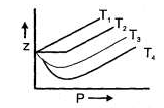Solution:
QUESTION: 6

In the Figure, isotherms of CO2 at several temperatures near the critical point are shown. At the critical point (critical state), the distinction between the liquid and gaseous states disappear and the density of the gaseous substance is equal to that in the liquid state. For every gas this occurs at specific values of temperature and pressure, called critical temperature and critical pressure respectively. At temperatures and pressures above the critical point value, a gas is said be in a supercritical state. Critical constants are evaluated by solving the Vander W aals equation which is a cubic in volume. The values are TcIn the supercritical region the behaviour is studied by plotting Z vs P plots. The variation in this region is studied with respect to boyles temperature aAt temperature
higher than CB the variation is linear (Plot I) and at temperature lower than CB the variation is non linear (Plot II) With reference to the passage answers the following questions:

Q.

A gas can be condensed to liquid through the paths I, II, and Ill, as shown in figure. The path(s) through which the gas changes to liquid abruptly is (are)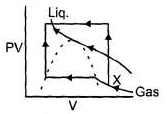Solution:
QUESTION: 7

Sketch shows the plot of Z v/s P for of a hypothetical gas for one mole at three distinct temperature.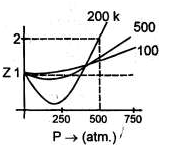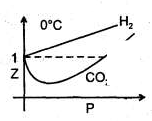Boyle’s temperature is the temperature at which a gas shows ideal behaviour over a pressure range in the low pressure region. Boyle's temperature (Tb) = a/Rb . If a plot is obtained at temperatures well below Boyle's temperature then the curve will show negative deviation, in low pressure region and positive deviation in the high pressure region. Near critical temperature the curve is more likely as CO2 and the temperature well above critical temperature curve is more like H2 at 0°C as shown above. At high pressure suppose all the constant temperature curve varies linearly with pressure according to the following equation: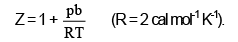Q.

Which of the following is correct:

Solution: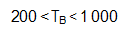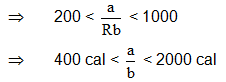QUESTION: 8

Sketch shows the plot of Z v/s P for of a hypothetical gas for one mole at three distinct temperature.Boyle’s temperature is the temperature at which a gas shows ideal behaviour over a pressure range in the low pressure region. Boyle's temperature (Tb) = a/Rb . If a plot is obtained at temperatures well below Boyle's temperature then the curve will show negative deviation, in low pressure region and positive deviation in the high pressure region. Near critical temperature the curve is more likely as CO2 and the temperature well above critical temperature curve is more like H2 at 0°C as shown above. At high pressure suppose all the constant temperature curve varies linearly with pressure according to the following equation:Q.

For 500 K plot value of Z changes from 2 to 2.2 if pressure is varied from 1000 atm to 1200 atm (high pressure) then the value of b/RTwill be

Solution:

In the high pressure region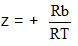solve for the two points.

QUESTION: 9

Sketch shows the plot of Z v/s P for of a hypothetical gas for one mole at three distinct temperature.Boyle’s temperature is the temperature at which a gas shows ideal behaviour over a pressure range in the low pressure region. Boyle's temperature (Tb) = a/Rb . If a plot is obtained at temperatures well below Boyle's temperature then the curve will show negative deviation, in low pressure region and positive deviation in the high pressure region. Near critical temperature the curve is more likely as CO2 and the temperature well above critical temperature curve is more like H2 at 0°C as shown above. At high pressure suppose all the constant temperature curve varies linearly with pressure according to the following equation:Q.

As shown in the figure at 200 K and 500 atm value of compressibility factor is 2 (approx). Then volume of the gas at this point will be

Solution: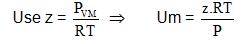QUESTION: 10

Sketch shows the plot of Z v/s P for of a hypothetical gas for one mole at three distinct temperature.Boyle’s temperature is the temperature at which a gas shows ideal behaviour over a pressure range in the low pressure region. Boyle's temperature (Tb) = a/Rb . If a plot is obtained at temperatures well below Boyle's temperature then the curve will show negative deviation, in low pressure region and positive deviation in the high pressure region. Near critical temperature the curve is more likely as CO2 and the temperature well above critical temperature curve is more like H2 at 0°C as shown above. At high pressure suppose all the constant temperature curve varies linearly with pressure according to the following equation:Q.

Plot at Boyle's temperature for the gas will be

Solution:

At boyles temp, a real gas shows ideal behaviour in the low pressure region.

QUESTION: 11

In very high pressure region if Z v/s P is plotted at 1200 K for the above gas then it will have greatest slope.

Solution: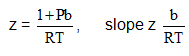and hence is inversely proportional to T.

QUESTION: 12

Match the following: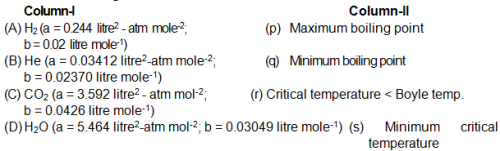Solution:
QUESTION: 13

For a fixed amount of the gas match the two column:

Column-I       Column II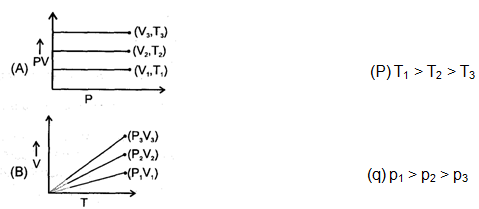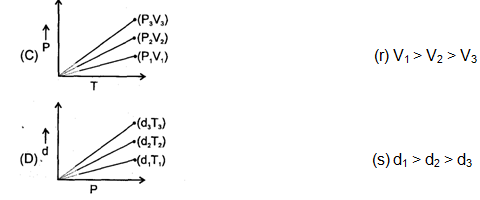Solution:
QUESTION: 14

Match the following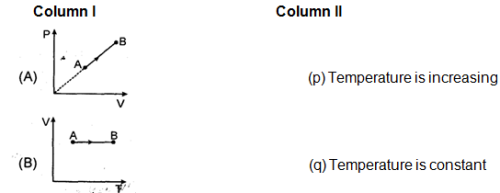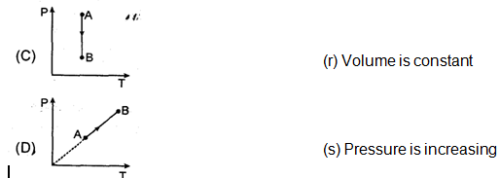Solution:
QUESTION: 15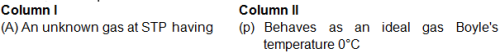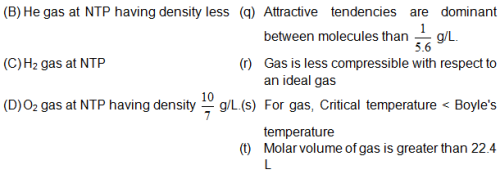Solution: